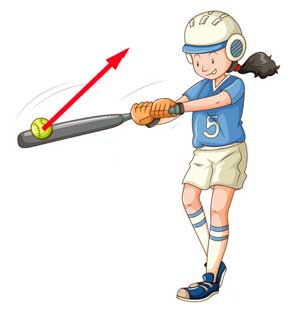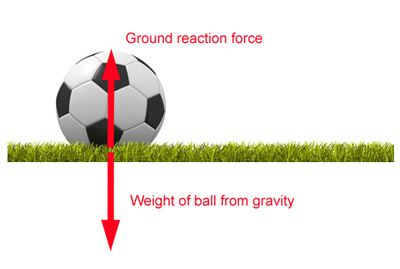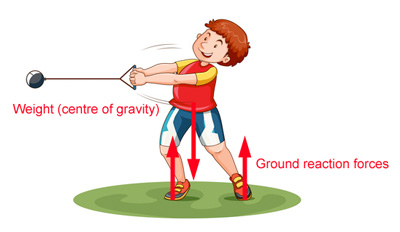A force is a push or a pull which alters the state of motion of a body and is measured in Newtons (N). As explained in Newton's first law of motion a force is required to make a stationary body move, change speed, direction or stop.

In sport and internal force is one which is generated within the body, for example, the leg muscles contracting to move the bones. An external force is one which acts outside the body, for example, the boxers glove as it strikes the head of their opponent.

Here are some examples of forces acting on a baseball player. Force is a vector quantity which means it needs BOTH magnitude (size) and direction. To get a better understanding of the forces acting in a particular situation we can draw arrows to indicate the direction the forces are acting. To do this we need three pieces of information:

• The point which the force is applied.
• The direction of the force.
• The size or magnitude of the force.The red arrow in the diagram above indicates the point at which the force acts, the direction and the size. If the force was bigger we could use a larger arrow.

## Net force

The net force is the sum of all the forces acting on a body. If the forces are balanced then the body will not move or change velocity. If the forces are not balanced then the body will move in the direction that the sum of the forces (all of them added up) acts.

## Balanced forces

In the diagram below the ball is stationary because the forces acting on it are balanced.## Unbalanced forces

In the diagram below the forces on the blue fighter are not balanced. The force of foot on the face is much greater than any resisting forces from the muscles of the blue fighter. Friction forces on the ground prevent the blue player from sliding. Gravity also acts on both fighters.

## Verticle forces

The forces acting on sports performers are weight (mass x acceleration from gravity) and the ground reaction force when the athlete is in contact with the ground. In the diagram below the verticle forces are weight acting downwards from the centre of gravity and the ground reaction forces acting up at the contact points of the feet. There are other rotational forces acting here but we are only considering the verticle forces for now.Related Articles

### Impulse

Impulse is simply a measure of the force applied for a specific time. Impulse = force x time and has units Ns (Newton seconds). Impulse is important in sport because many techniques, particularly...

### Free Body Diagrams

Free body diagrams are used to show which forces are acting on a body at a particular instant in time. Arrows indicate the position, direction and size of the force acting. The most likely forces...